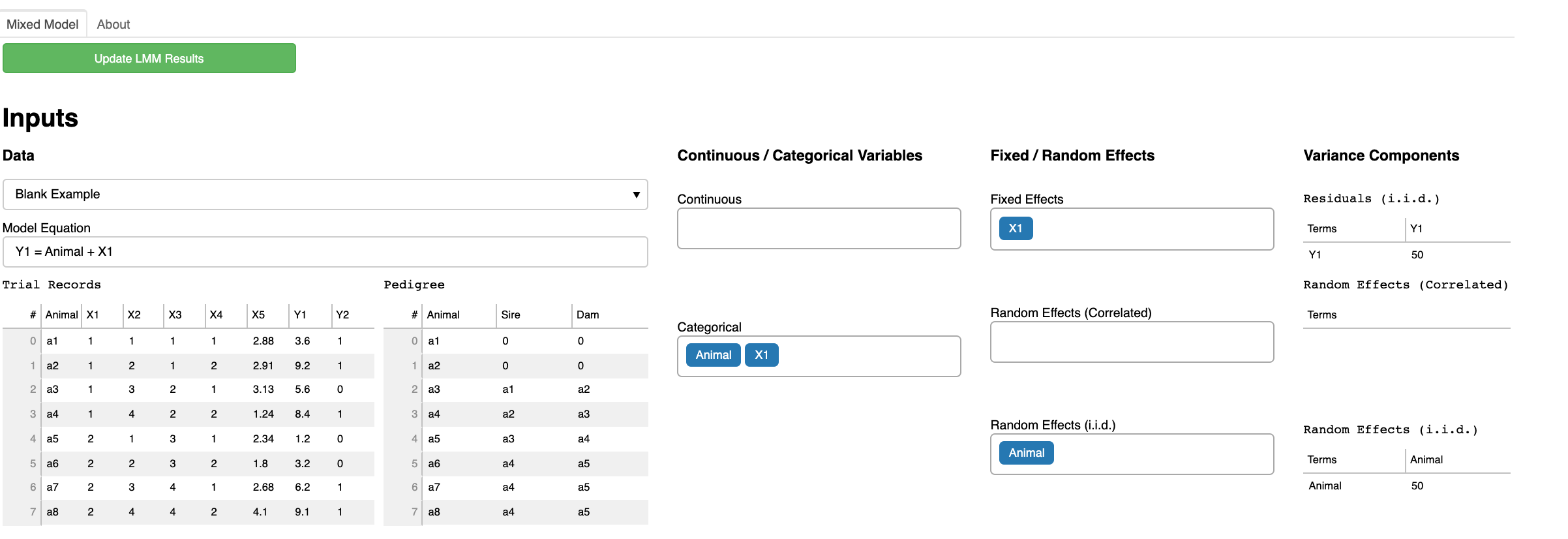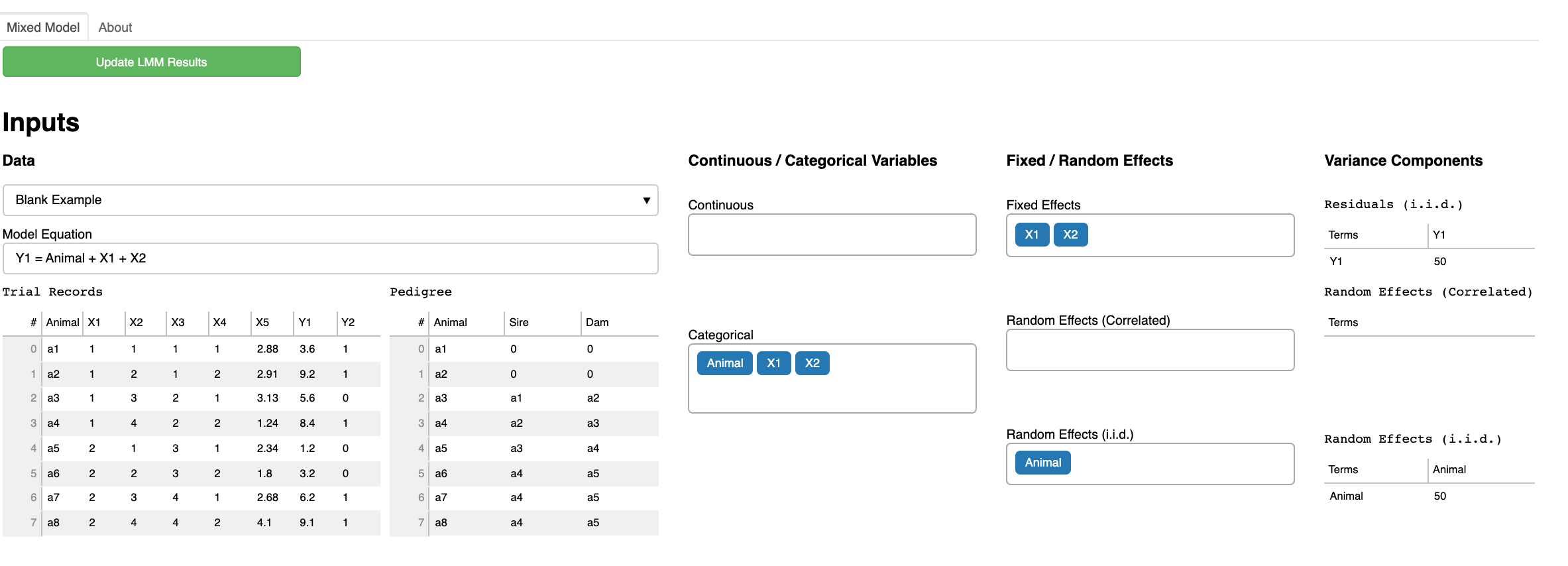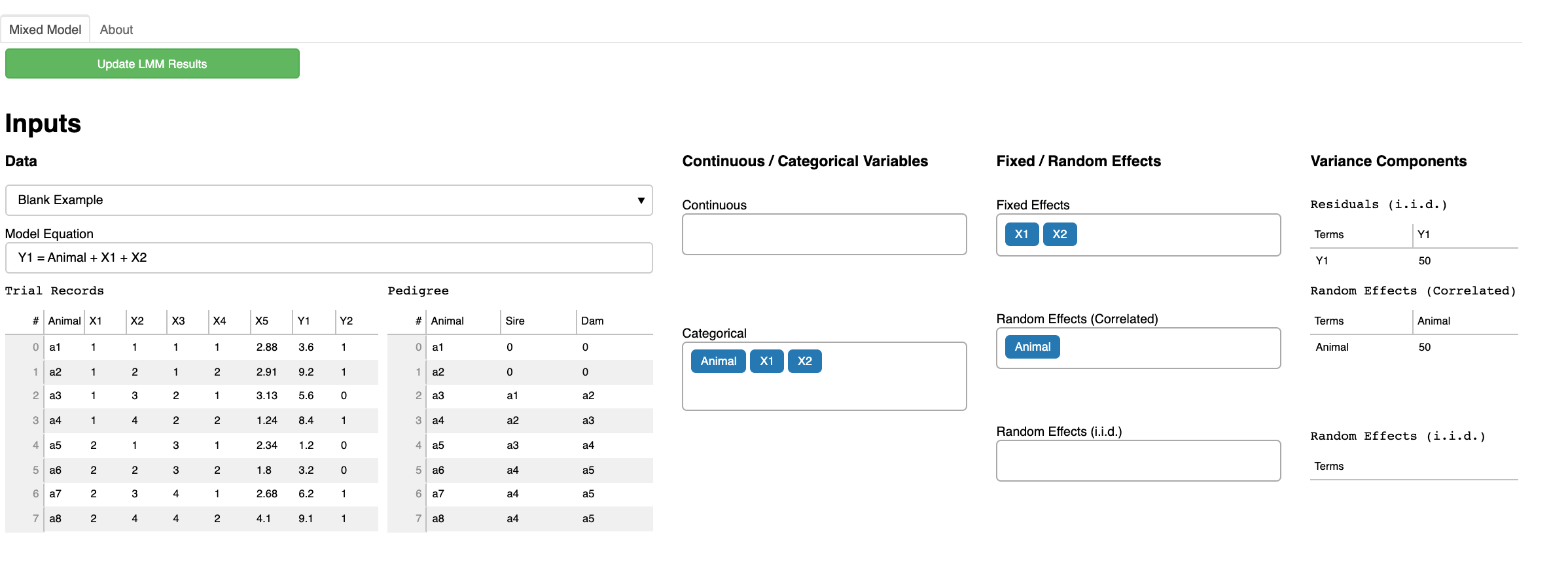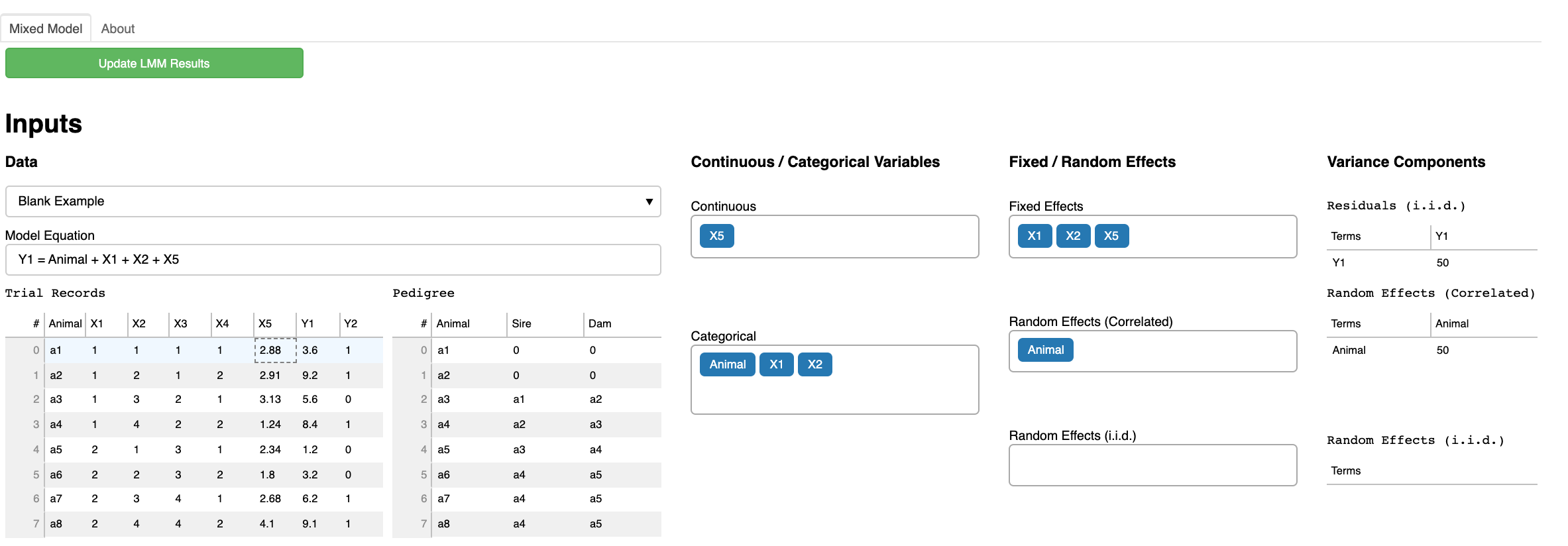# High-throughput Phenotyping Driven Quantitative Genetics @CMA-FCT-NOVA

## Background

This is the day 1 computer lab session for quantitative genetic analysis. The goal of this exercise is to understand mixed model equations (MME) using an interactive MME visualization tool LMMonBoard.

## Exercise 1

1. Select Blank Example from the Data drop-down list.
2. In Model equation, remove + X2 + X5 so that you have Y1 = Animal + X1.
3. In the Fixed / Random Effects column, select Animal from the Random Effects (i.i.d.) drop-down list.
4. Click Update LMM Results on the right upper corner.Assume that the residual variance $$\sigma^2_e = 1$$ and the additive genetic variance $$\sigma^2_g = 1$$.

### Questions

• How many fixed effects are in the model? How many levels?
• How many random effects are in the model? How many levels?
• Interpret each component of MME.

## Exercise 2

1. In Model equation, add + X2 so that you have Y1 = Animal + X1 + X2.
2. In the Fixed / Random Effects column, select Animal from the Random Effects (i.i.d.) drop-down list.
3. Click Update LMM Results on the right upper corner.### Questions

• How many fixed effects are in the model? How many levels?
• How many random effects are in the model? How many levels?
• Interpret each component of MME.

## Exercise 3

1. In the Fixed / Random Effects column, select Animal from the Random Effects (Correlated) drop-down list.
2. Click Update LMM Results on the right upper corner.### Questions

• How many fixed effects are in the model? How many levels?
• How many random effects are in the model? How many levels?
• Interpret each component of MME.

## Exercise 4

1. In Model equation, add + X5 so that you have Y1 = Animal + X1 + X2 + X5.
2. In the Fixed / Random Effects column, select Animal from the Random Effects (Correlated) drop-down list.
3. In the Continuous / Categorical Variables column, select X5 from the Continuous drop-down list.
4. Click Update LMM Results on the right upper corner.### Questions

• How many fixed effects are in the model? How many levels?
• How many random effects are in the model? How many levels?
• Interpret each component of MME.